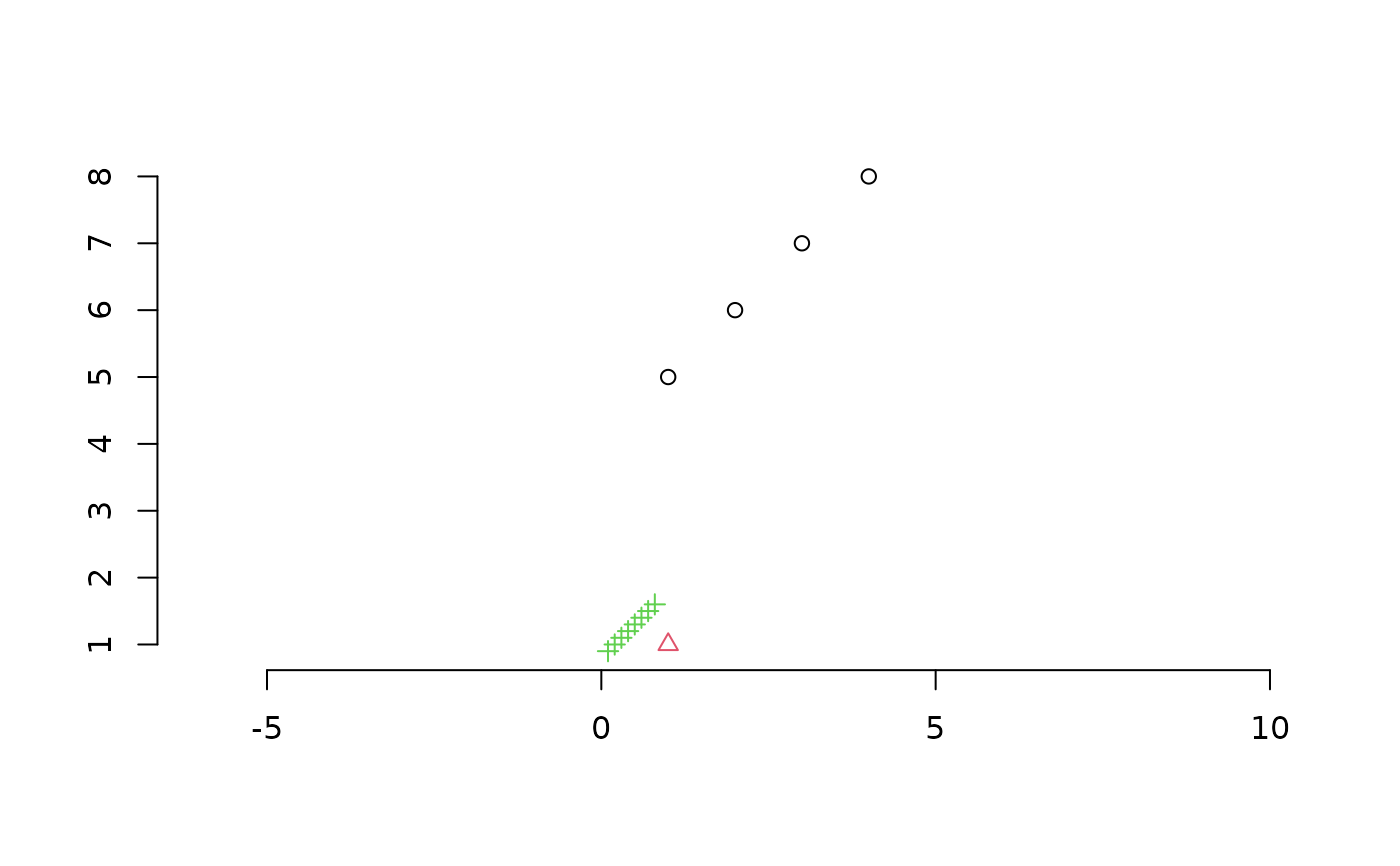Cluster size statistics

SumOfRanges(x, cluster = 1)

SumOfVariances(x, cluster = 1)

SumOfVars(x, cluster = 1)

MeanCentroidDistance(x, cluster = 1)

MeanCentDist(x, cluster = 1)

MeanCentroidDist(x, cluster = 1)

DistanceFromMedian(x, cluster = 1)

DistFromMed(x, cluster = 1)

MeanNN(x, cluster = 1)

MeanMSTEdge(x, cluster = 1)

## Arguments

x

Matrix in which each row lists the coordinates of a point in a Euclidian space; or, where supported, dist object specifying distances between each pair of points.

cluster

Optional integer vector specifying the cluster or group to which each row in x belongs.

## Value

SumOfRanges() returns a numeric specifying the sum of ranges within each cluster across all dimensions.

SumOfVariances() returns a numeric specifying the sum of variances within each cluster across all dimensions.

MeanCentroidDistance() returns a numeric specifying the mean distance from the centroid to points in each cluster.

DistanceFromMedian() returns a numeric specifying the mean distance of each point (except the median) from the median point of its cluster.

MeanNN() returns a numeric specifying the mean distance from each point within a cluster to its nearest neighbour.

MeanMSTEdge() returns a numeric specifying the mean length of an edge in the minimum spanning tree of points within each cluster.

Other tree space functions: MSTSegments(), MapTrees(), MappingQuality(), SpectralEigens(), median.multiPhylo()

Other cluster functions: KMeansPP()

## Examples

points <- rbind(matrix(1:16, 4), rep(1, 4), matrix(1:32, 8, 4) / 10)
cluster <- rep(1:3, c(4, 1, 8))

plot(
points[, 1:2], # Plot first two dimensions of four-dimensional space
col = cluster, pch = cluster, # Style by cluster membership
asp = 1, # Fix aspect ratio to avoid distortion
ann = FALSE, frame = FALSE # Simple axes
)SumOfRanges(points, cluster)
#>  12.0  0.0  2.8
SumOfVariances(points, cluster)
#>  6.666667       NA 0.240000
MeanCentroidDistance(points, cluster)
#>  2.0 0.0 0.4
DistanceFromMedian(points, cluster)
#>  2.6666667        NA 0.4571429
MeanNN(points, cluster)
#>  2.0  NA 0.2
MeanMSTEdge(points, cluster)
#>  2.0  NA 0.2